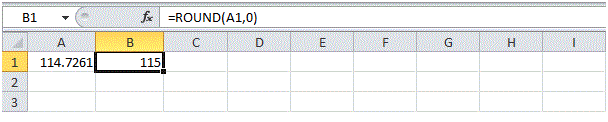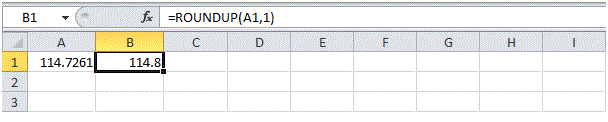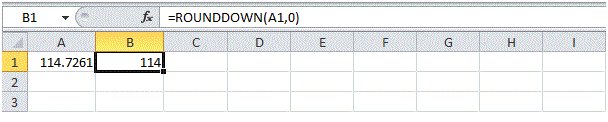# The ROUND, ROUNDUP and ROUNDDOWN function in Excel

## The ROUND, ROUNDUP and ROUNDDOWN function in MS Excel

The ROUND function is used to round the fraction values into a rounded figure to a specified number of digits. This function is very important and has many implementation scenarios.

There are three functions to round numbers in MS Excel. The ROUND, ROUNDUP and ROUNDDOWN function.

## ROUND

Round a number to two decimal places.In the example above, 114.7261 get rounded up to 114.73.

Round a number to one decimal place.Round a number to the nearest integer.Round a number to the nearest 10.In the above example, the integer value is further rounded to the nearest 10.

Round a number to the nearest 100.In the above example, the integer value is further rounded to the nearest 100.

## ROUNDUP

The ROUNDUP function is used to rounds a number up regardless if it is near to 0. In the example below, you will observe that the value in cell A1 is rounded up to 1 fractional digit.## ROUNDDOWN

The ROUNDDOWN function is used to rounds a number downward regardless if it is near to 10. In the example below, you will observe that the value in cell A1 is rounded down to its integer value.